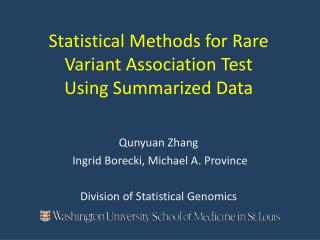# Statistical Methods for Rare Variant Association Test Using Summarized Data - PowerPoint PPT PresentationDownload PresentationStatistical Methods for Rare Variant Association Test Using Summarized Data

Statistical Methods for Rare Variant Association Test Using Summarized DataDownload Presentation## Statistical Methods for Rare Variant Association Test Using Summarized Data

- - - - - - - - - - - - - - - - - - - - - - - - - - - E N D - - - - - - - - - - - - - - - - - - - - - - - - - - -
##### Presentation Transcript

1. Statistical Methods for Rare Variant Association Test Using Summarized Data Qunyuan Zhang Ingrid Borecki, Michael A. Province Division of Statistical Genomics

2. Next generation sequencing => rare variants Two types of data Motivation Summarized level • Pooled DNA sequencing • Public data (as control) Individual level

3. Existing Methods

4. EFTTFT QQ Plots of Existing Methods(under the null) EFT and C-alpha inflated with false positives TFT and CAST no inflation, but assuming single effect-direction Objective More general, powerful methods … CAST C-alpha

5. variant 1 variant 2 … … variant 3 variant k variant i Structure of Summarized data Strategy Instead of testing total freq./number, we test the randomness of all tables.

6. Exact Probability Test (EPT) 1.Calculating the probability of each table based on hypergeometric distribution 2. Calculating the logarized joint probability (L) for all k tables 3. Enumerating all possible tables and L scores 4. Calculating p-value P= Prob.( )

7. Likelihood Ratio Test (LRT) Binomial distribution

8. EPT N=500 LRT N=500 Q-Q Plots of EPT and LRT(under the null) LRT N=3000 EPT N=3000

9. Power Comparison significance level=0.00001 Variant proportion Positive causal 80% Neutral 20% Negative Causal 0% Power Power Power Sample size Sample size Sample size

10. Power Comparisonsignificance level=0.00001 Variant proportion Positive causal 60% Neutral 20% Negative Causal 20% Power Sample size

11. Power Comparison significance level=0.00001 Variant proportion Positive causal 40% Neutral 20% Negative Causal 40% Power Sample size

12. Power Comparison individual-level data vs. summarized dataN=1000, significance level=0.00001 Power CMC Li & Leal, 2008 SKAT Wu et al., 2011 Variant proportion positive : neutral : negative (%)

13. Cases: 460 ovarian cancer cases, germline exome data, from TCGA Controls: ~3500 individuals, exome data, from NHBLI Application -LOG10 p-values of 933 cancer-related genes

14. Conclusions EFT and C-alpha produce inflated p-value. TFT and CAST produce correct p-value, but lose power in detecting bi-directional effects. EPT produces correct p-value and maintains power regardless of effect directions, more computer time. LRT produces slightly biased p-value for small N, can be improved by larger N, similar power of EPT, less computer time, a good alternative for large datasets. If no confounders need to be modeled, there is no significant loss of power in the use of summarized data

15. Acknowledgements Dr. Li Ding Charles Lu Krishna-Latha Kanchi (for providing the TCGA and NHBLI exome data)